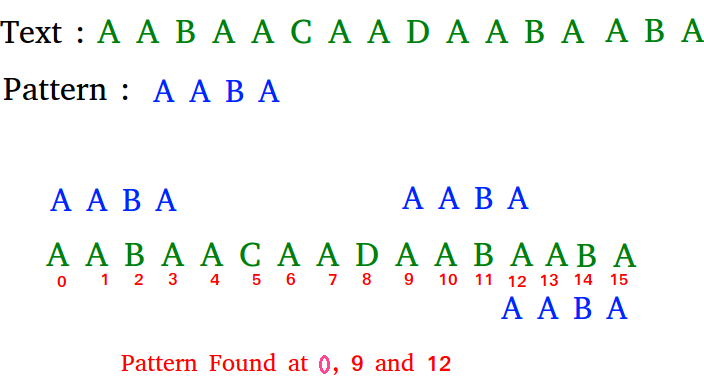# C Program for KMP Algorithm for Pattern Searching

Given a text txt[0..n-1] and a pattern pat[0..m-1], write a function search(char pat[], char txt[]) that prints all occurrences of pat[] in txt[]. You may assume that n > m.

Examples:

```Input:  txt[] = "THIS IS A TEST TEXT"
pat[] = "TEST"
Output: Pattern found at index 10

pat[] =  "AABA"
Output: Pattern found at index 0
Pattern found at index 9
Pattern found at index 12```

## Recommended: Please solve it on “PRACTICE ” first, before moving on to the solution.

Pattern searching is an important problem in computer science. When we do search for a string in notepad/word file or browser or database, pattern searching algorithms are used to show the search results.

## C++

 `// C++ program for implementation of KMP pattern searching ` `// algorithm ` `#include ` ` `  `void` `computeLPSArray(``char``* pat, ``int` `M, ``int``* lps); ` ` `  `// Prints occurrences of txt[] in pat[] ` `void` `KMPSearch(``char``* pat, ``char``* txt) ` `{ ` `    ``int` `M = ``strlen``(pat); ` `    ``int` `N = ``strlen``(txt); ` ` `  `    ``// create lps[] that will hold the longest prefix suffix ` `    ``// values for pattern ` `    ``int` `lps[M]; ` ` `  `    ``// Preprocess the pattern (calculate lps[] array) ` `    ``computeLPSArray(pat, M, lps); ` ` `  `    ``int` `i = 0; ``// index for txt[] ` `    ``int` `j = 0; ``// index for pat[] ` `    ``while` `(i < N) { ` `        ``if` `(pat[j] == txt[i]) { ` `            ``j++; ` `            ``i++; ` `        ``} ` ` `  `        ``if` `(j == M) { ` `            ``printf``(``"Found pattern at index %d "``, i - j); ` `            ``j = lps[j - 1]; ` `        ``} ` ` `  `        ``// mismatch after j matches ` `        ``else` `if` `(i < N && pat[j] != txt[i]) { ` `            ``// Do not match lps[0..lps[j-1]] characters, ` `            ``// they will match anyway ` `            ``if` `(j != 0) ` `                ``j = lps[j - 1]; ` `            ``else` `                ``i = i + 1; ` `        ``} ` `    ``} ` `} ` ` `  `// Fills lps[] for given patttern pat[0..M-1] ` `void` `computeLPSArray(``char``* pat, ``int` `M, ``int``* lps) ` `{ ` `    ``// length of the previous longest prefix suffix ` `    ``int` `len = 0; ` ` `  `    ``lps = 0; ``// lps is always 0 ` ` `  `    ``// the loop calculates lps[i] for i = 1 to M-1 ` `    ``int` `i = 1; ` `    ``while` `(i < M) { ` `        ``if` `(pat[i] == pat[len]) { ` `            ``len++; ` `            ``lps[i] = len; ` `            ``i++; ` `        ``} ` `        ``else` `// (pat[i] != pat[len]) ` `        ``{ ` `            ``// This is tricky. Consider the example. ` `            ``// AAACAAAA and i = 7. The idea is similar ` `            ``// to search step. ` `            ``if` `(len != 0) { ` `                ``len = lps[len - 1]; ` ` `  `                ``// Also, note that we do not increment ` `                ``// i here ` `            ``} ` `            ``else` `// if (len == 0) ` `            ``{ ` `                ``lps[i] = 0; ` `                ``i++; ` `            ``} ` `        ``} ` `    ``} ` `} ` ` `  `// Driver program to test above function ` `int` `main() ` `{ ` `    ``char` `txt[] = ``"ABABDABACDABABCABAB"``; ` `    ``char` `pat[] = ``"ABABCABAB"``; ` `    ``KMPSearch(pat, txt); ` `    ``return` `0; ` `} `

Output:

```Found pattern at index 10
```

Please refer complete article on KMP Algorithm for Pattern Searching for more details!

My Personal Notes arrow_drop_up
Article Tags :

Be the First to upvote.

Please write to us at contribute@geeksforgeeks.org to report any issue with the above content.